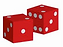Why Games Are So Important

We all want children to be fluent in their basic math facts and we want them to be able to recall facts quickly and accurately. But before we rush to memorization, we need to develop what is called  “number sense” which is the ability to see connections and visualize the basic facts.

Using ten frames, strategies like knowing and using doubles, and using families of facts
all promote number sense.

Playing games with our children is a  great for practicing recall and gaining speed.
“Worksheets” on the other hand are not fun and sadly many online games are just electronic worksheets.

A two-player game fosters discussion around the math facts and develops good social skills .

Go Fish to Make Ten (Grade 1)

A two player game using an ordinary deck of cards to develop fluency in the pairs of number 1 to 9 that "make ten".

##### (Video)A two player game using an ordinary deck of cards to develop fluency in adding the numbers 1 to 10.

##### (Video)A two player game using an ordinary deck of cards to develop fluency in subtracting the numbers 1 to 10.

Subtraction War Directions (PDF)

##### (Video)

A two player game using an ordinary deck of cards to develop fluency in the multiplying the numbers 1 to 10.

##### (Video)A two player game using dice to develop fluency in adding the numbers 1 to 6.

##### ​

(Video)A two player game using dice to develop fluency in multiplying the numbers 1 to 6.

##### (Video)